Courses

# Ex 8.1 NCERT Solutions- Quadrilaterals Class 9 Notes | EduRev

## Mathematics (Maths) Class 9

Created by: Full Circle

## Class 9 : Ex 8.1 NCERT Solutions- Quadrilaterals Class 9 Notes | EduRev

The document Ex 8.1 NCERT Solutions- Quadrilaterals Class 9 Notes | EduRev is a part of the Class 9 Course Mathematics (Maths) Class 9.
All you need of Class 9 at this link: Class 9

Question 1. The angles of quadrilateral are in the ratio 3 : 5 : 9 : 13. Find all the angles of the quadrilateral.
Solution:
Let the angles of the quadrilateral be 3x, 5x, 9x and 13x.
∵ Sum of all the angles of quadrilateral = 360º
∴ 3x + 5x + 9x + 13x = 360º ⇒ 30x = 360º
⇒ x=(360o/30)= 12°
∴  3x = 3 x 12° = 36º 5x
= 5 x 12° = 60º 9x = 9 x 12°
= 108º 13x = 13 x 12° = 156º
⇒ The required angles of the quadrilateral are 36º, 60º, 108º and 156º.

Question 2. If the diagonals of a parallelogram are equal, then show that it is a rectangle.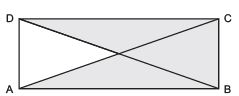Solution: A parallelogram ABCD such that AC = BD
In ΔABC and ΔDCB, AC = DB             [Given]
AB = DC            [Opposite sides of a parallelogram]
BC = CB            [Common]
ΔABC ≌ ΔDCB             [SSS criteria]
∴ Their corresponding parts are equal.
⇒ ∠ABC = ∠DCB            …(1)
∵ AB || DC and BC is a transversal.             [∵ ABCD is a parallelogram]
∴ ∠ABC + ∠DCB = 180º [Interior opposite angles are supplementary] …(2)
From (1) and (2), we have ∠ABC = ∠DCB = 90º
i.e. ABCD is parallelogram having an angle equal to 90º.
∴ ABCD is a rectangle.

Question 3. Show that if the diagonals of a quadrilateral bisect each other at right angles, then it is a rhombus.
Solution:
We have a quadrilateral ABCD such that the diagonals AC and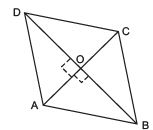BD bisect each other at right angles at O.
∴ In ΔAOB and ΔAOD, we have AO = AO            [Common]
OB = OD            [Given that O in the mid-point of BD]
∠AOB = ∠AOD            [Each = 90°]
∴ ΔAOB ≌ ΔAOD        [SAS criteria]

⇒ Their corresponding parts are equal. ∴ AB = AD …(1)
Similarly, AB = BC …(2) BC = CD …(3)
∴ From (1), (2), (3) and (4),
we have AB = BC = CD = DA Thus, the quadritateral ABCD is a rhombus.

Question 4. Show that the diagonals of a square are equal and bisect each other at right angles.
Solution:
We have a square ABCD such that its diagonals AC and BD intersect at O.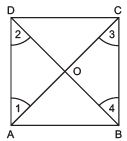(i) To prove that the diagonals are equal, i.e. AC = BD In ΔABC and ΔBAD, we have
AB = BA             [Common]
BC = AD            [Opposite sides of the square ABCD]
∠ABC = ∠BAD            [All angles of a square are equal to 90º]
∴ DABC ≌ DBAD             [SAS criteria]
⇒ Their corresponding parts are equal.
⇒ AC = BD            …(1)

(ii) To prove that ‘O’ is the mid-point of AC and BD.
∵ AD || BC and AC is a transversal.             [∵ Opposite sides of a square are parallel]
∴ ∠1 = ∠3            [Interior alternate angles]
Similarly, ∠2= ∠4            [Interior alternate angles]
Now, in ΔOAD and ΔOCB, we have AD = CB            [Opposite sides of the square ABCD]
∠1= ∠3            [Proved] ∠2= ∠4            [Proved]
∴ ΔOAD ≌ ΔOCB            [ASA criteria]
∴ Their corresponding parts are equal.
⇒ OA = OC and OD = OB ⇒ O is the mid-point of AC and BD,
i.e. the diagonals AC and BD bisect each other at O.            …(2)

(iii) To prove that AC ⊥ BD.
In ΔOBA and ΔODA, we have OB = OD             [Proved]
BA = DA            [Opposite sides of the square]
OA = OA             [Common]
∴ ΔOBA ≌ ΔODA            [SSS criteria]
⇒ Their corresponding parts are equal.
⇒ ∠AOB = ∠AOD
But ∠AOB and ∠AOD form a linear pair.
∴ ∠AOB + ∠AOD = 180º
⇒ ∠AOB = ∠AOD = 90º
⇒ AC ⊥ BD             …(3)
From (1), (2) and (3), we get AC and BD are equal and bisect each other at right angles.

Question 5. Show that if the diagonals of a quadrilateral are equal and bisect each other at right angles, then it is a square.
Solution:
We have a quadrilateral ABCD such that ‘O’ is the mid-point of AC and BD. Also AC ⊥ BD.
Now, in ΔAOD and ΔAOB, we have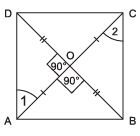AO = AO             [Common]
OD = OB            [∵ O is the mid-point of BD]
∠AOD = ∠AOB             [Each = 90º]
∴ ΔAOD ≌ ΔAOB            [SAS criteria]
∴ Their corresponding parts are equal.
Similarly, we have AB = BC             …(2)
BC = CD             …(3)
CD = DA             …(4)
From (1), (2), (3) and (4) we have: AB = BC = CD = DA
∴ Quadrilateral ABCD is having all sides equal.
In ΔAOD and ΔCOB, we have AO = CO            [Given]
OD = OB            [Given]
∠AOD = ∠COB            [Vertically opposite angles]
∴ ΔAOD ≌ ΔCOB
⇒ Their corresponding parts are equal. ⇒ ∠1= ∠2 But, they form a pair of interior alternate angles.
Similarly, AB || DC
∴ ABCD is a parallelogram.
∵ Parallelogram having all of  its sides equal is a rhombus.
∴ ABCD is a rhombus.
Now, in ΔABC and ΔBAD, we have AC = BD            [Given]
AB = BA            [Common]
∴ ΔABC ≌ ΔBAD [SSS criteria]
⇒ Their corresponding angles are equal.
Since, AD || BC and AB is a transversal.
∴ ∠ABC + ∠BAD = 180º             [Interior opposite angles are supplementary]
i.e. The rhombus ABCD is having one angle equal to 90º.
Thus, ABCD is a square.

Question 6. Diagonal AC of a parallelogram ABCD bisects ∠ A (see figure). Show that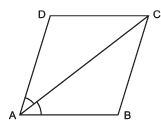(i) it bisects ∠ C also, (ii) ABCD is a rhombus.
Solution:
We have a parallelogram ABCD in which diagonal AC bisects ∠A.
⇒ ∠DAC = ∠BAC

(i) To prove that AC bisects ∠C.
∵ ABCD is a parallelogram.
∴ AB || DC and AC is a transversal.
∴ ∠1 = ∠3[Alternate interior angles]             …(1)
Also, BC || AD and AC is a transversal.
∴ ∠2 = ∠4[Alternate interior angles]            …(2)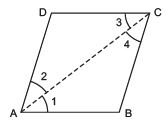But AC bisects ∠A.            [Given]
∴ ∠1 = ∠2            …(3)
From (1), (2) and (3), we have ∠3= ∠4
⇒ AC bisects ∠C.

(ii) To prove ABCD is a rhombus.
In DABC, we have ∠1= ∠4[∵ ∠1 = ∠2 = ∠4]
⇒ BC = AB            [Sides opposite to equal angles are equal]             …(4)
But ABCD is a parallelogram            [Given]
∴ AB = DC            [Opposite sides of a parallelogram]            …(6)
From (4), (5) and (6), we have AB = BC = CD = DA
Thus, ABCD is a rhombus.

Question 7. ABCD is a rhombus. Show that diagonal AC bisects ∠A as well as ∠C and diagonal BD bisects ∠B as well as ∠D.
Solution: ABCD is a rhombus.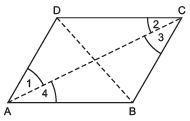∴ AB = BC = CD = AD
Also, AB || CD an AD || BC
Now, AD = CD ⇒ ∠1 = ∠2             …(1)
[Angles opposite to equal sides are equal]
Also, CD || AB             [Opposite sides of the parallelogram] and AC is AC is transversal.
∴ ∠1= ∠3            [Alternate interior angles]            …(2)
From (1) and (2), we have ∠2= ∠3 and ∠1 = ∠4 ⇒ AC bisects ∠C as well as ∠A.
Similarly, we prove that BD bisects ∠B as well as ∠D.

Question 8. ABCD is a rectangle in which diagonal AC bisects ∠ A as well as ∠ C. Show that: (i) ABCD is a square (ii) diagonal BD bisects ∠ B as well as ∠ D.
Solution:
We have a rectangle ABCD such that AC bisects ∠ A as well as ∠C.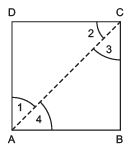i.e. ∠ 1= ∠ 4 and ∠ 2 = ∠ 3             ...(1)

(i) Since, rectangle is a parallelogram.
∴ ABCD is a parallelogram.
⇒ AB || CD and AC is a transversal.
∴ ∠2= ∠4            [Alternate interior angles] .            ..(2)
From (1) and (2), we have ∠3= ∠4
⇒ AB = BC             [∵ Sides opposite to equal angles in D ABC are equal.]
∴ AB = BC = CD = AD
⇒ ABCD is a rectangle having all of its sides equal.
∴ ABCD is a square.

(ii) Since, ABCD is a square, and diagonals of a square bisect the opposite angles.
∴ BD bisects ∠B as well as ∠D.

Question 9. In parallelogram ABCD, two points P and Q are taken on diagonal BD such that DP = BQ (see figure). Show that: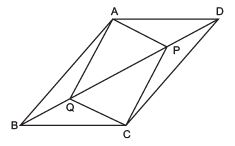(i) ΔAPD ≌ ΔCQB (ii) AP = CQ (iii) ΔAQB ≌ ΔCPD (iv) AQ = CP (v) APCQ is a parallelogram.
Solution:
We have parallelogram ABCD. BD is a diagonal and ‘P’ and ‘Q’ are such that
PD = QB            [Given]

(i) To prove that ΔAPD ≌ ΔCQB
∵ AD || BC and BD is a transversal.            [∵ ABCD is a parallelogram.]
∴ ∠ADB = ∠CBD            [Interior alternate angles]
Now, in ΔAPD and ΔCQB, we have
AD = CB            [Opposite side of the parallelogram]
P D = QB            [Given]
∴ Using SAS criteria, we have ΔAPD ≌ ΔCQB

(ii) To prove that AP = CQ Since ΔAPD ≌ ΔCQB            [Proved]
∴ Their corresponding parts are equal. ⇒ AP = CQ

(iii) To prove that ΔAQB ≌ ΔCPD.
∵ AB || CD and BD is a transversal.            [∵ ABCD is a parallelogram.]
∴ ∠ABD = ∠CDB
⇒ ∠ABQ = ∠CDP Now, in ΔAQB and ΔCPD,
we have QB = PD            [Given]
∠ABQ = ∠CDP            [Proved]
AB = CD            [Opposite sides of parallelogram ABCD]
∴ ΔAQB ≌ ΔCPD            [SAS criteria]

(iv) To prove that AQ = CP.
Since ΔAQB ≌ ΔCPD            [Proved]
∴ Their corresponding parts are equal.
⇒ AQ = CP.

(v) To prove that APCQ is a parallelogram.
Let us join AC.
Since, the diagonals of a || gm bisect each other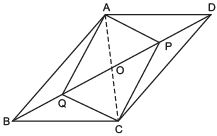∴ AO = CO …(1)
and BO = DO
⇒ (BO ∠ BQ) = (DO ∠ DP)            [∵ BQ = DP            (Given)]
⇒ QO = PO            …(2)
Now, in quadrilateral APCQ, we have AO = CO and QO = PO
[from (1) and (2)]
i.e. AC and QP bisect each other at O.
⇒ APCQ is a parallelogram.

Question 10. ABCD is a parallelogram and AP and CQ are perpendiculars from vertices A and C on diagonal BD (see figure).
Show that (i)
ΔAPB ≌ ΔCQD (ii) AP = CQ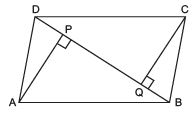Solution: (i) In ΔAPB and ΔCQD, we have
∠APB = ∠CQD [90º each] AB = CD

[Opposite sides of parallelogram ABCD]
∠ABP = ∠CDQ            [AB || CD and AB is a transversal]
⇒ Using AAS criteria, we have ΔAPB ≌ ΔCQD

(ii) Since, ΔAPB ≌ ΔCQD             [Proved]
∴ Their corresponding parts are equal.
⇒ AP = CQ

Example 11. In D ABC and D DEF, AB = DE, AB || DE, BC = EF and BC || EF. Vertices A, B and C are joined to vertices D, E and F respectively (see figure). Show that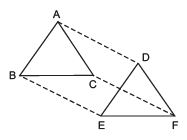(i) quadrilateral ABED is a parallelogram.

(ii) quadrilateral BEFC is a parallelogram.

(iv) quadrilateral ACFD is a parallelogram.

( v ) AC = DF (vi) D ABC ≌ D DEF.
Solution:
(i) To prove that ABED is a parallelogram.
Since “A quadrilateral is a parallelogram if a pair of opposite sides is parallel and is of equal length.” Now AB = DE [Given]
AB | | DE            [Given]
i.e. ABED is a quadrilateral in which a pair of opposite sides (AB and DE) is parallel and of equal length.
∴ ABED is a parallelogram.

(ii) To prove that BECF is a parallelogram.
∵ BC = EF            [Given]
and BC || EF            [Given]
i.e. BECF is a quadrilateral in which a pair of opposite sides (BC and EF) is parallel and of equal length.
∴ BECF is a parallelogram.

(iii) To prove that AD || CF and AD = CF ∵ ABED is a parallelogram. [Proved]
∴ Its opposite sides are parallel and equal.
Also BEFC is a parallelogram.             [Proved]
∴ BE || CF and BE = CF            [∵ Opposite sides of a parallelogram are parallel and equal]         …(2)

From (1) and (2), we have AD || CF and AD = CF

(iv) To prove that ACFD is a parallelogram.
i.e. In quadrilateral ACFD, one pair of opposite sides (AD and CF) is parallel and of equal length.
∴ Quadrilateral ACFD is a parallelogram.

(v) To prove that AC = DE.
∵ ACFD is a parallelogram.            [Proved]
∴ Its opposite sides are parallel and of equal length. i.e. AC = DF

(vi) To prove that ΔABC ≌ ΔDEF In D ABC and DDEF, we have:
AB = DE            [Opposite sides of a parallelogram]
BC = EF            [Opposite sides of a parallelogram]
AC = DF            [Proved]
∴ Using SSS criteria, we have ΔABC ≌ ΔDEF.

Question 12. ABCD is a trapezium in which AB || CD and AD = BC (see figure).
Show that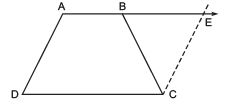(i) ∠ A = ∠ B (ii) ∠ C = ∠ D (iii) ∠ ABC ≌ ∠ BAD (iv) Diagonal AC = Diagonal BD Hint: Extend AB and draw a line through C parallel to DA intersecting AB produced at E.
Solution:
We have AB || CD and AD = BC (i) To prove that ∠A = ∠B.
Produce AB to E and draw CE || AD.

∴ AB || DC
⇒ AE || DC            [Given]
∴ AECD is a parallelogram.
⇒ AD = CE             [opposite sides of the parallelogram AECD]
∴ BC = CE
Now, in ΔBCE, we have BC = CE
⇒ ∠CBE = ∠CEB            …(1)
[∵ Angles opposite to equal sides of a triangle are equal]
Also, ∠ABC + ∠CBE = 180°            [Linear pair]               ...(2)
and ∠A + ∠CEB = 180°            [∵ Adjacent angles of a parallelogram are supplementary]         …(3)
From (2) and (3), we get ∠ABC + ∠CBE = ∠A + ∠CEB
But ∠CBE = ∠CEB             [Using (1)]
∴ ∠ABC = ∠A
or ∠B= ∠A
or ∠A= ∠B

(ii) To prove that ∠C = ∠D.
AB || CD and AD is a transversal.
∴ ∠A + ∠D = 180º            [Sum of interior opposite angles]
Similarly, ∠B + ∠C = 180º
⇒ ∠A + ∠D= ∠B + ∠C
But ∠A= ∠B            [Proved]
∴ ∠C= ∠D

(iii) To prove ΔABC ≌ ΔBAD In ΔABC and ΔBAD, we have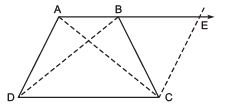AB = BA            [Common]
∴ ΔABC ≌ ΔBAD [Using SAS criteria]

(iv) To prove that diagonal AC = diagonal BD ∵
∴ Their corresponding parts are equal.
⇒ the diagonal AC = the diagonal BD.

,

,

,

,

,

,

,

,

,

,

,

,

,

,

,

,

,

,

,

,

,

;